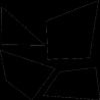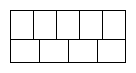#### You may also like### Framed

Seven small rectangular pictures have one inch wide frames. The frames are removed and the pictures are fitted together like a jigsaw to make a rectangle of length 12 inches. Find the dimensions of the pictures.### Tilted Squares

It's easy to work out the areas of most squares that we meet, but what if they were tilted?### From One Shape to Another

Read about David Hilbert who proved that any polygon could be cut up into a certain number of pieces that could be put back together to form any other polygon of equal area.

# Four or Five

##### Age 11 to 14 ShortChallenge Level

The diagram shows a large rectangle composed of nine identical smaller rectangles.Both the length and breadth of each of these smaller rectangles are whole numbers of centimetres. Which of the following could be the area of the large rectangle?

a. 450 square centimetres
b. 1260 square centimetres
c. 1620 square centimetres

If you liked this problem, here is an NRICH task which challenges you to use similar mathematical ideas.
This problem is taken from the UKMT Mathematical Challenges.
You can find more short problems, arranged by curriculum topic, in our short problems collection.